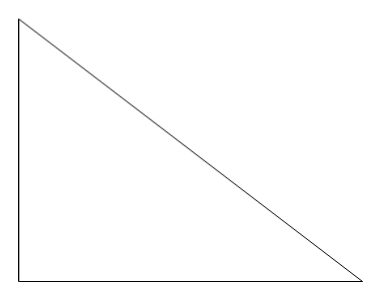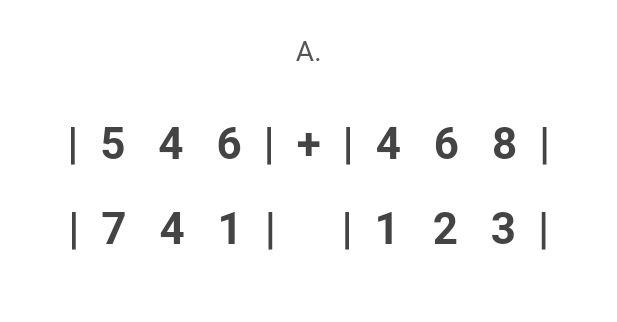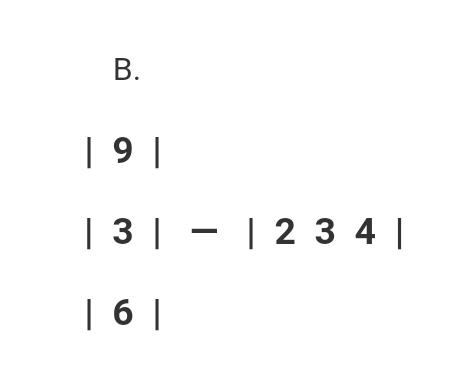# Third Term Examination Mathematics SS 2 – Exam Questions

Last Updated on June 13, 2021 by Alabi M. S.

[mediator_tech]

### SECTION A

1. Express 0.00349 in a standard form.

(a) 34.9 x 10^-4

(b) 3.49 x 10^-3

(c) 0.003 x 10^-3

(d) 349 x 10^-5

Use the frequency table below to answer questions 2 – 4.

Scores                15      20     25      30      35

Frequency           5        4       2        7        2

2. Find the mean of the scores.

(a) 5

(b) 7

(c) 25

(d) 4

3. Find the median of the scores.

(a) 5

(b) 7

(c) 25

(d) 4

4. What is the mode of the scores?

(a) 5

[mediator_tech]

(b) 7

(c) 25

(d) 4

5. ____________ means uncertainty.

(a) Discount

(b) Probability

(c) Dimension

(d) Construction

6. ……………………………………………………………………….

(a) x ≤ 3

(b) x < 3

(c) x ≥ 3

(d) x > 3

7. 2x + 3x + 58° + 90° + 12° = 360°. Find x.

(a) 15

(b) 12

(c) 20

(d) 10

8. The sum of two angles equals 90° is called ____________.

(a) supplementary

(b) complementary

(c) opposite

(d) transversal

9. Convert 42two to base ten.

(a) 5410

[mediator_tech]

(b) 4510

(c) 4710

(d) 5010

10. In ABC, A = 29°, B = 36°, B = 15.8cm. Find a.

(a) 13.0cm

(b) 13.1cm

(c) 0.02cm

(d) 15.2cm

11. All are solid shapes except ____________.

(a) cuboids

(b) cube

(c) triangle

(d) pyramid

12. Angle of elevation is the angle the observer stands to look ____________.

(a) up

(b) down

(c) right

(d) left

13. ____________ are made up of rows and columns.

(a) Vertices

(b) Modules

(c) Matrices

(d) Probability

14. Simplify: 3/4 + 31/5 – 5/6.

(a) 31/30

[mediator_tech]

(b) 31/15

(c) 31/60

(d) 31/12

15. The length of arc is calculated using ____________.

(a) Ө/360 x 2Л²

(b) Ө/360 x Л²

(c) Ө/360 x 2Лr²

(d) Ө/360 x 2Лrh

16. A box of 20 balls (4 red, 12 green, 4 yellow). What is the probability of picking red?

(a) 1/5

(b) 3/5

(c) 2/5

(d) 4/10

17. What is the probability of picking green ball?

(a) 1/5

(b) 3/5

(c) 2/5

(d) 4/10

18. The area of sector is calculated using ____________.

(a) Ө/360 x 2Лr

(b) Ө/360 x Лr²

(c) Ө/360 x 2Лr²

(d) Ө/360 x 2Лrh

19. ____________ is the area of a cylinder.

(a) Лr²

[mediator_tech]

(b) 2Лr²

(c) 2Лr

(d) Лr²h

20. Find the area of cuboid of 5cm long, 6cm wide and 7cm deep.

(a) 210cm

(b) 30cm

(c) 35cm

(d) 240cm

### SECTION B

QUESTION 1

A. Differentiate between the two triangles below using angle of elevation, angle of depression and observer’s view.B. State the volume of:

I. Cube

II. Cuboid

III. Cylinder

QUESTION 2

A. Calculate the length of arc of angle 70° and radius of 7cm.

[mediator_tech]

B. Find the radius of length of arc of 21cm and angle of 60°.

C. Calculate the volume of the shape given:QUESTION 3

There are 50 shoes in a carton; 10 blue shoes, 10 red shoes, 15 black shoes, 5 white shoes and 10 green shoes. When picked randomly, what is the probability of picking.

A. Red shoe

B. Green shoe

C. Neither white nor black shoe

D. Either blue or green shoe

E. Grey shoe

QUESTION 4

Solve the following matrices:[mediator_tech]